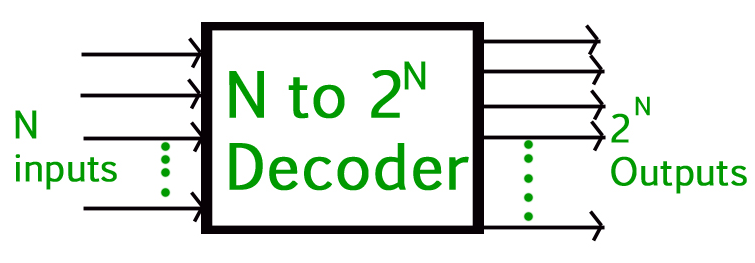Open in App
Not now

# GATE | GATE CS 2020 | Question 30

• Last Updated : 27 Sep, 2021

If there are m input lines n output lines for a decoder that is used to uniquely address a byte addressable 1 KB RAM, then the minimum value of m+n is ________ .

Note – This question was Numerical Type.
(A) 18
(B) 1034
(C) 10
(D) 1024

Explanation: According to Decoder:We need 210 outputs to map 1 KB RAM. For this we need 10 × 210 decoder.
Therefore,

`Here m = 10 and n = 210 `

Hence,

`m + n = 10 + 210 = 10 + 1024 = 1034  `

Option (B) is correct.

My Personal Notes arrow_drop_up
Related Articles